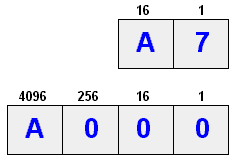(redirected from Hexdecimal numbering system)
Also found in: Dictionary, Thesaurus, Medical.
Related to Hexdecimal numbering system: octal number system

a number system having a base 16; the symbols for the numbers 0--9 are the same as those used in the decimal system, and the numbers 10--15 are usually represented by the letters A--F. The system is used as a convenient way of representing the internal binary code of a computer
Collins Discovery Encyclopedia, 1st edition © HarperCollins Publishers 2005

[‚hek·sə′des·məl]
(mathematics)
Pertaining to a number system using the base 16. Also known as sexadecimal.
McGraw-Hill Dictionary of Scientific & Technical Terms, 6E, Copyright © 2003 by The McGraw-Hill Companies, Inc.

(mathematics)
(Or "hex") Base 16. A number representation using the digits 0-9, with their usual meaning, plus the letters A-F (or a-f) to represent hexadecimal digits with values of (decimal) 10 to 15. The right-most digit counts ones, the next counts multiples of 16, then 16^2 = 256, etc.

digit weight value B = 11 16^3 = 4096 11*4096 = 45056 E = 14 16^2 = 256 14* 256 = 3584 A = 10 16^1 = 16 10* 16 = 160 D = 13 16^0 = 1 13* 1 = 13 ----- BEAD = 48813

There are many conventions for distinguishing hexadecimal numbers from decimal or other bases in programs. In C for example, the prefix "0x" is used, e.g. 0x694A11.

Hexadecimal is more succinct than binary for representing bit-masks, machines addresses, and other low-level constants but it is still reasonably easy to split a hex number into different bit positions, e.g. the top 16 bits of a 32-bit word are the first four hex digits.

The term was coined in the early 1960s to replace earlier "sexadecimal", which was too racy and amusing for stuffy IBM, and later adopted by the rest of the industry.

Actually, neither term is etymologically pure. If we take "binary" to be paradigmatic, the most etymologically correct term for base ten, for example, is "denary", which comes from "deni" (ten at a time, ten each), a Latin "distributive" number; the corresponding term for base sixteen would be something like "sendenary". "Decimal" is from an ordinal number; the corresponding prefix for six would imply something like "sextidecimal". The "sexa-" prefix is Latin but incorrect in this context, and "hexa-" is Greek. The word octal is similarly incorrect; a correct form would be "octaval" (to go with decimal), or "octonary" (to go with binary). If anyone ever implements a base three computer, computer scientists will be faced with the unprecedented dilemma of a choice between two *correct* forms; both "ternary" and "trinary" have a claim to this throne.

## hex

(HEXadecimal) Hexadecimal means 16, and the base 16 numbering system is used as a shorthand for representing binary numbers. Each half byte (four bits) is assigned a hex digit or letter as in the following chart with its decimal and binary equivalents. Hex values are identified with an "h" or dollar sign, thus \$A7, A7h and A7H all stand for hex A7. See hex chart and hex editor.
```    Base16   10   2Hex  Dec  Binary
0    0    0000
1    1    0001
2    2    0010
3    3    0011
4    4    0100
5    5    0101
6    6    0110
7    7    0111
8    8    1000
9    9    1001
A   10    1010
B   11    1011
C   12    1100
D   13    1101
E   14    1110
F   15    1111
```

Interpreting the HexAs decimal digits increment by 10, hexadecimal digits increment by 16. Hexadecimal is a shorthand for human readability of binary, which is the intrinsic numbering system in the computer. See binary values.

``` A7
= decimal 167 (10x16+7x1)
= binary 10100111 (128+32+4+2+1)

A000
= decimal 40,960 (10x4096)
= binary 1010000000000000 (32768+8192)
```
Copyright © 1981-2019 by The Computer Language Company Inc. All Rights reserved. THIS DEFINITION IS FOR PERSONAL USE ONLY. All other reproduction is strictly prohibited without permission from the publisher.
Site: Follow: Share:
Open / Close